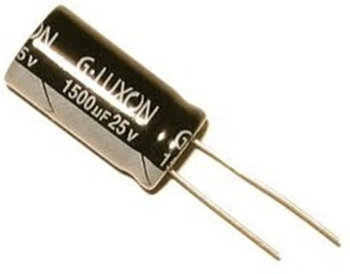﻿ RC Time Constant of a CapacitorRC Time Constant (τ) of a CapacitorThe RC Time Constant (τ) of a Capacitor is the amount of time it takes for a capacitor to charge to 63% of the supply voltage which is charging it. For capacitors that are fully charged, the RC time constant is the amount of time it takes for a capacitor to discharge to 63% of its fully charged voltage.

The formula to calculate the time constant is:

Time Constant (τ)=RC

The unit for the time constant is seconds (s). R stands for the resistance value of the resistor and C is the capacitance of the capacitor.

The Time Constant is affected by two variables, the resistance of the resistor and the capacitance of the capacitor. The larger any or both of the two values, the longer it takes for a capacitor to charge or discharge. If the resistance is larger, the capacitor takes a longer time to charge, because the greater resistance creates a smaller current. If the capacitance of the capacitor is a larger value, the capacitor takes a longer time to charge because it holds a larger charge, therefore, it takes longer to fill up. And, conversely, the smaller the resistance and capacitor values, the shorter time it takes for a capacitor to charge or discharge.

Example

Below we have a circuit of a 9-volt battery charging a 1000µF capacitor through a 3KΩ resistor:One time constant, τ=RC=(3KΩ)(1000µF)=3 seconds. So after 3 seconds, the capacitor is charged to 63% of the 9 volts that the battery is supplying it, which would be approximately 5.67 volts.

If R=1KΩ and C=1000µF, the time constant of the circuit is τ=RC=(1KΩ)(1000µF)=1 second.

If R=330KΩ and C=0.05µF, the time constant of the circuit is τ=RC=(330KΩ)(0.05µF)=16.5ms.

Related Resources